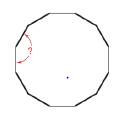Chapter 2.5, Problem 39EElementary Geometry For College St...

7th Edition
Alexander + 2 others
ISBN: 9781337614085

Solutions

Chapter
SectionElementary Geometry For College St...

7th Edition
Alexander + 2 others
ISBN: 9781337614085
Textbook Problem

The face of a clock has the shape of a regular polygon with 12 sides. What is the measure of the angle formed by two consecutive sides?To determine

To find:

The measure of the angle formed by two consecutive sides of a clock has the shape of a regular polygon with 12 sides.

Explanation

Given:

The following figure shows the given clock.

The given clock is a regular polygon of 12 sides.

Formula used:

The formula to calculate the angle formed by two consecutive sides of a n sided regular polygon is given by,

I=(n2)180°n

Here, I denotes each interior angle.

Calculation:

Substitute 12 for n in the above mentioned formula to obtained the angle formed by two consecutive sides of a clock

Still sussing out bartleby?

Check out a sample textbook solution.

See a sample solution

The Solution to Your Study Problems

Bartleby provides explanations to thousands of textbook problems written by our experts, many with advanced degrees!

Get Started

let f(x) = x 1, g(x) = x+1, and h(x) = 2x3 1. Find the rule for each function. 11. fg

Applied Calculus for the Managerial, Life, and Social Sciences: A Brief Approach

Convert the expressions in Exercises 6584 to power form. 3

Finite Mathematics and Applied Calculus (MindTap Course List)

For

Study Guide for Stewart's Multivariable Calculus, 8th

The appropriate option for the value of the indefinite integral ∫sinx2 dx.

Study Guide for Stewart's Single Variable Calculus: Early Transcendentals, 8th